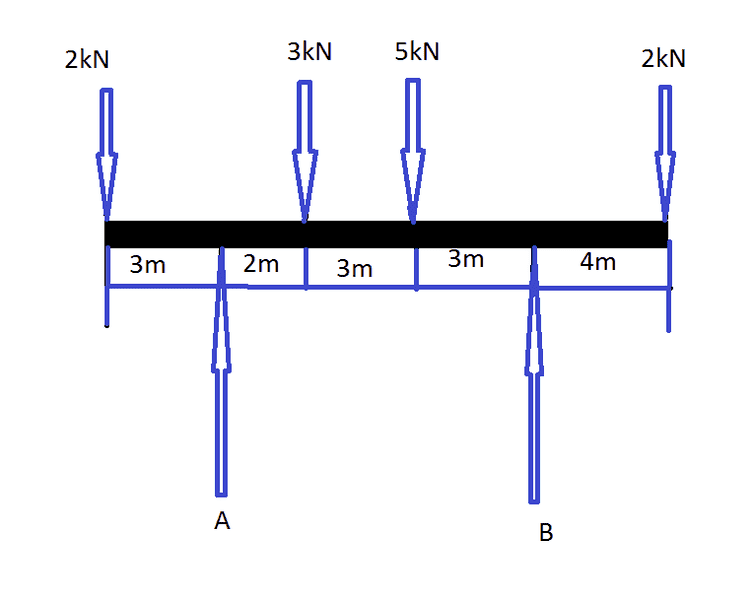# Solving Moments of Force: Finding A & B

• isigne

#### isigne

1.How do I find the force of A and B. I am taking moments at A but I am not getting the correct answer. (More Details below of attempt)

## Homework Equations

Ʃ Of Clockwise Moments = Ʃ Of Anti-Clockwise Moments
Ʃ Of Upward Force = Ʃ Of Downward Force

## The Attempt at a Solution

(THIS IS WRONG BUT THIS IS MY ATTEMPT)

A => (3x2)+(5x5)+(12x2)= Bx11
=> 6 + 25 + 24 = B x 11
=> 55 = B x 11
=> 55/11 = B
=> 5 = b

THANKS FOR HELP, I was trying this weekend and I need some genius advice so when I get this question again, I will be able to do so :)

A => (3x2)+(5x5)+(12x2)= Bx11
I assume you're using A as your pivot point.
(1) You neglected the moment due to the 2kN force at the left end.
(2) What's the distance between B and A?